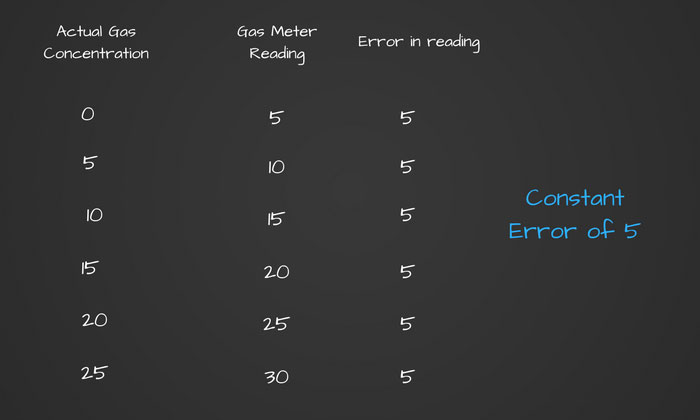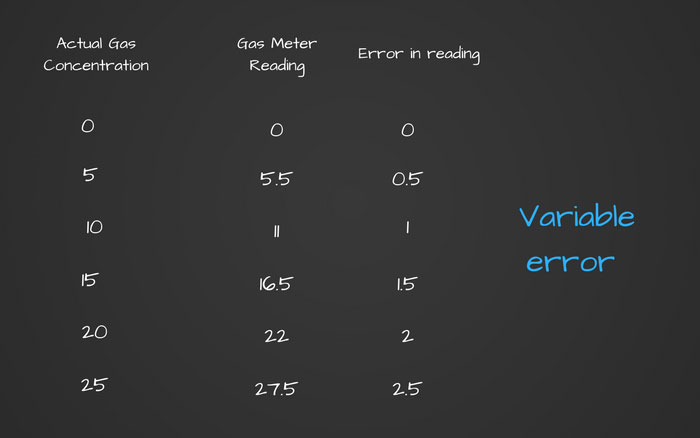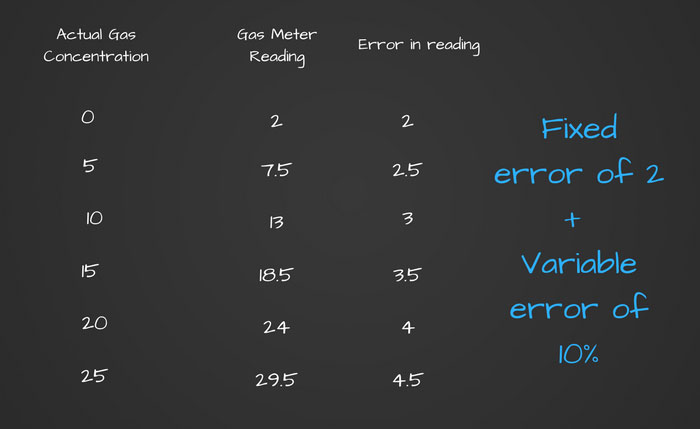# Q.Why do we need to zero calibrate the multi-gas meter? Does it affect its calibration with gas bottles?

Calibration with gas bottles is called span correction and I will use that term in this answer.

Let us understand the zero correction and span correction in gas meters. Let us say that we get following readings at various levels or concentration of a gas.You would see that in this case we have a constant error of 5.

And this error is because zero of the gas meter is not matching and that error in zero is being carried to all other readings. This is the zero error.

Now say in fresh air when gas meter should show zero reading instead of 5, we correct the gas meter reading to zero.

You would notice that all the other readings will also start showing correctly because we have corrected the constant error (zero error) that the gas meter had.

Now take this second example.You would notice that the error is not constant but is increasing as the gas concentration value increases. You would also note that in this case, the error is 10% of the actual gas concentration.

In this case, if we correct any readings (for example when gas concentration is 25) with the span correction, all other readings will get automatically corrected.

Span correction will reduce all the readings by 10%. This would not affect zero reading because 10% of zero is zero itself.

Now finally take the third case.Here the gas meter has both the zero error as well as span error. In this case if we try to do the span correction first, the gas meter will still not show the correct reading.

First we need to correct the zero error of 2 and then do the span correction to correct the span error of 10%.

Here are the take away points regarding gas meters calibration.

• Zero calibration corrects the zero error which is the fixed error in all the reading including at zero value.
• Span calibration corrects the variable error (increasing error with increase in value).
• Span correction does not affect the zero correction.
• While doing any calibration on gas meters, we first need to check any zero error and correct it. After we have corrected zero error, we can then check for span error and correct it if found.